« Vertical deformation of beams Final conclusion »

Torsional stiffness of beams

The effect of torsional stiffness of beams was studied parametrically as a percentage οf full elastic torsional stiffness of beams (100%, 10%, 1%). Similar results obtained from both finite element models (bar, two-dimensional). Ιn the case of full elastic torsional stiffness, both side beams are stressed by high torsion, caused by the moments on the supports of the main joists sitting on those beams. For 10% the torsion of the two beams is of moderate intensity, while for 1% both beams are practically not stressed by torsion.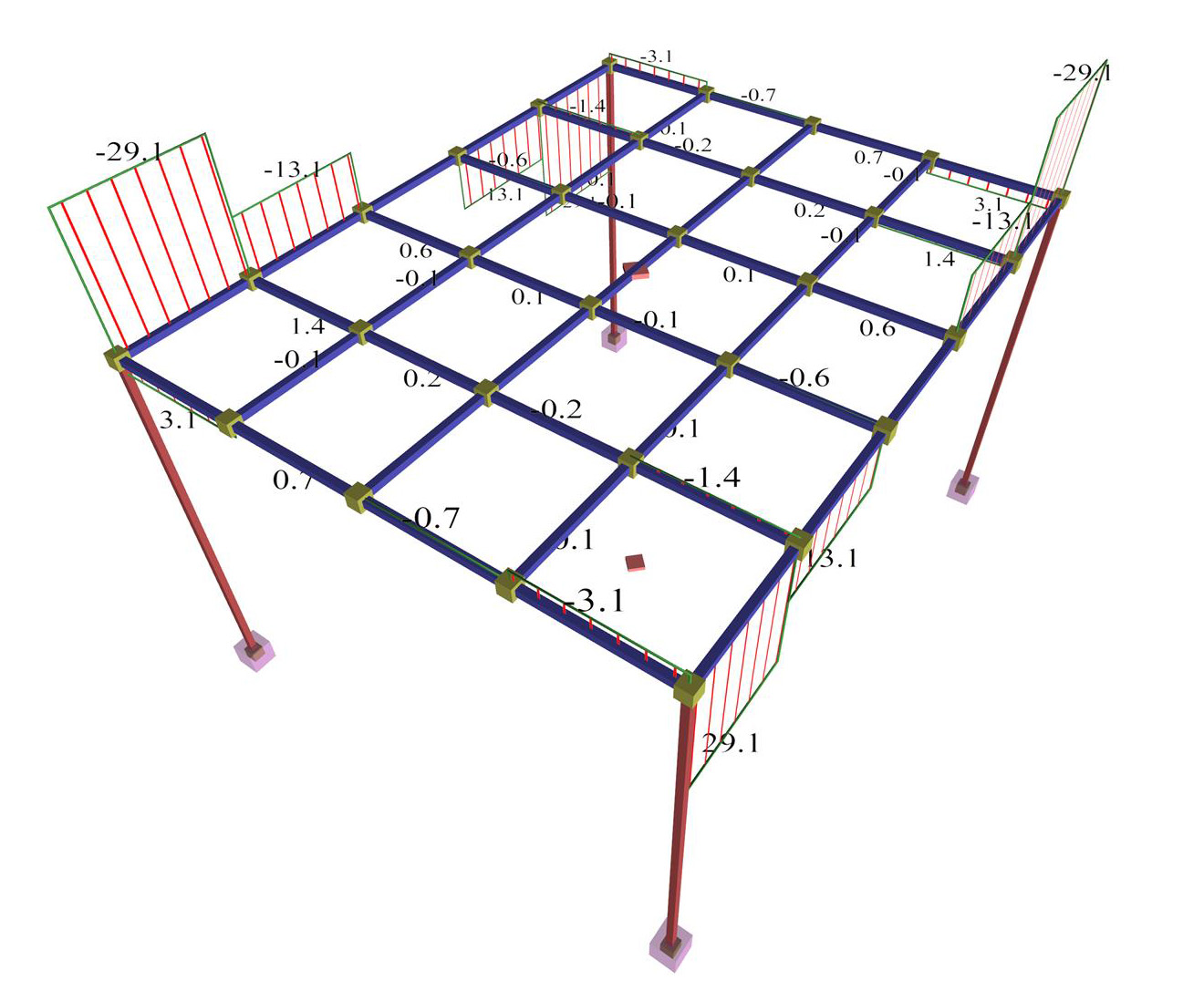Figure 3.3.6.3-1: Torsional moments of beams of torsional stiffness 100%. Bar finite element model Figure 3.3.6.3-1: Torsional moments of beams of torsional stiffness 100%. Bar finite element model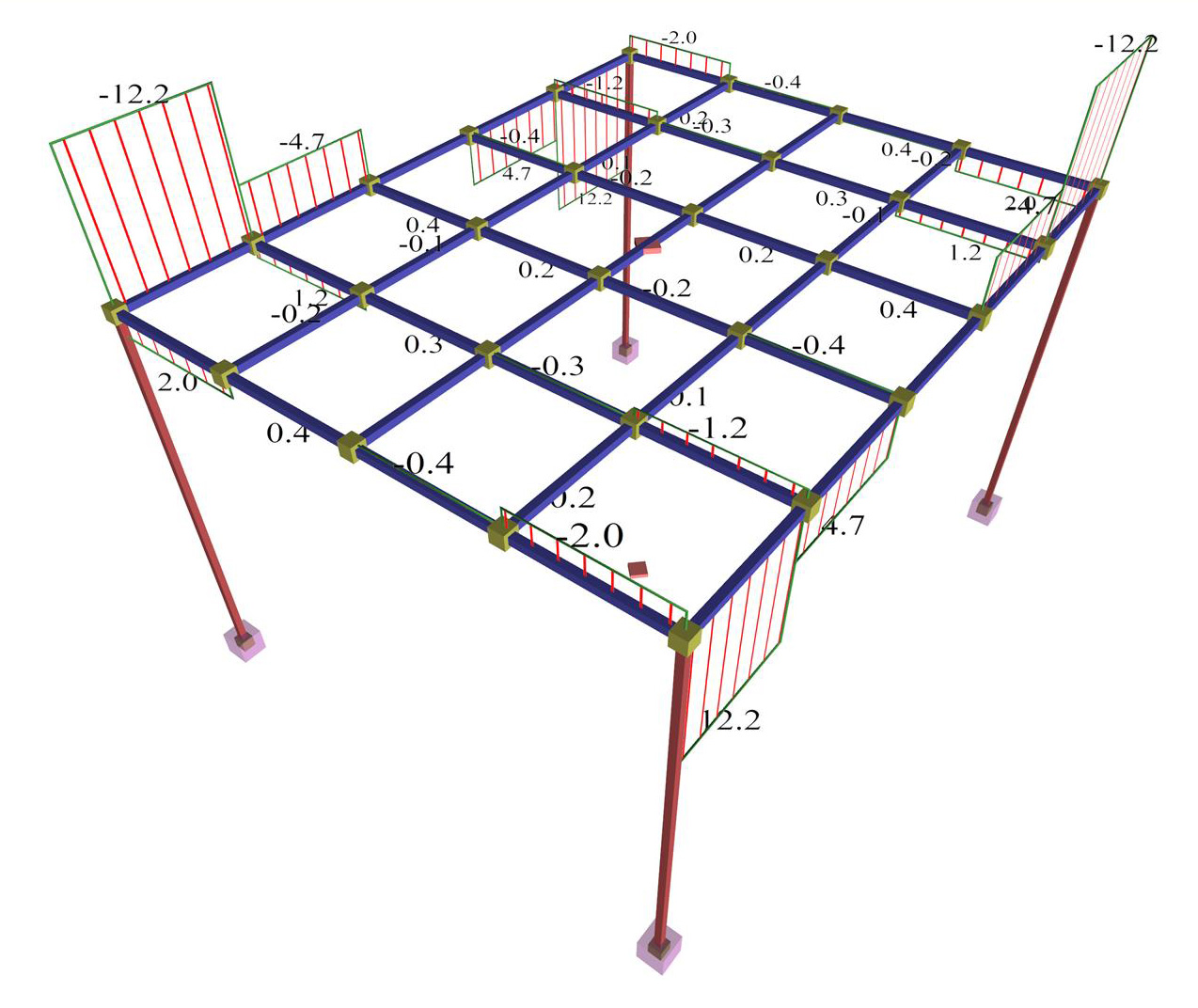Figure 3.3.6.3-2: Torsional moments of beams of torsional stiffness 10%. Bar finite element model Figure 3.3.6.3-2: Torsional moments of beams of torsional stiffness 10%. Bar finite element model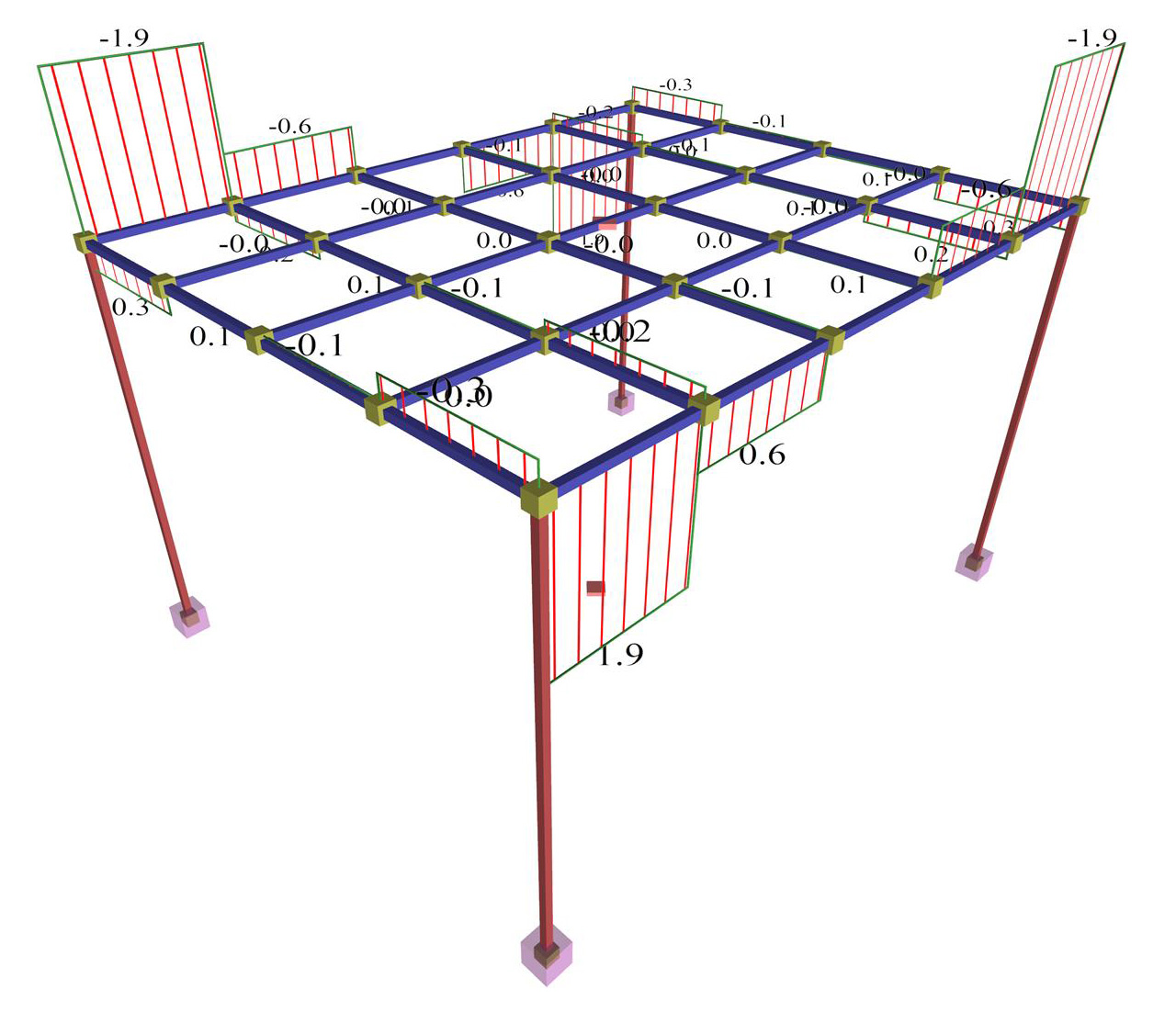Figure 3.3.6.3-3: Torsional moments of beams of torsional stiffness 1%. Bar finite element model. Figure 3.3.6.3-3: Torsional moments of beams of torsional stiffness 1%. Bar finite element model.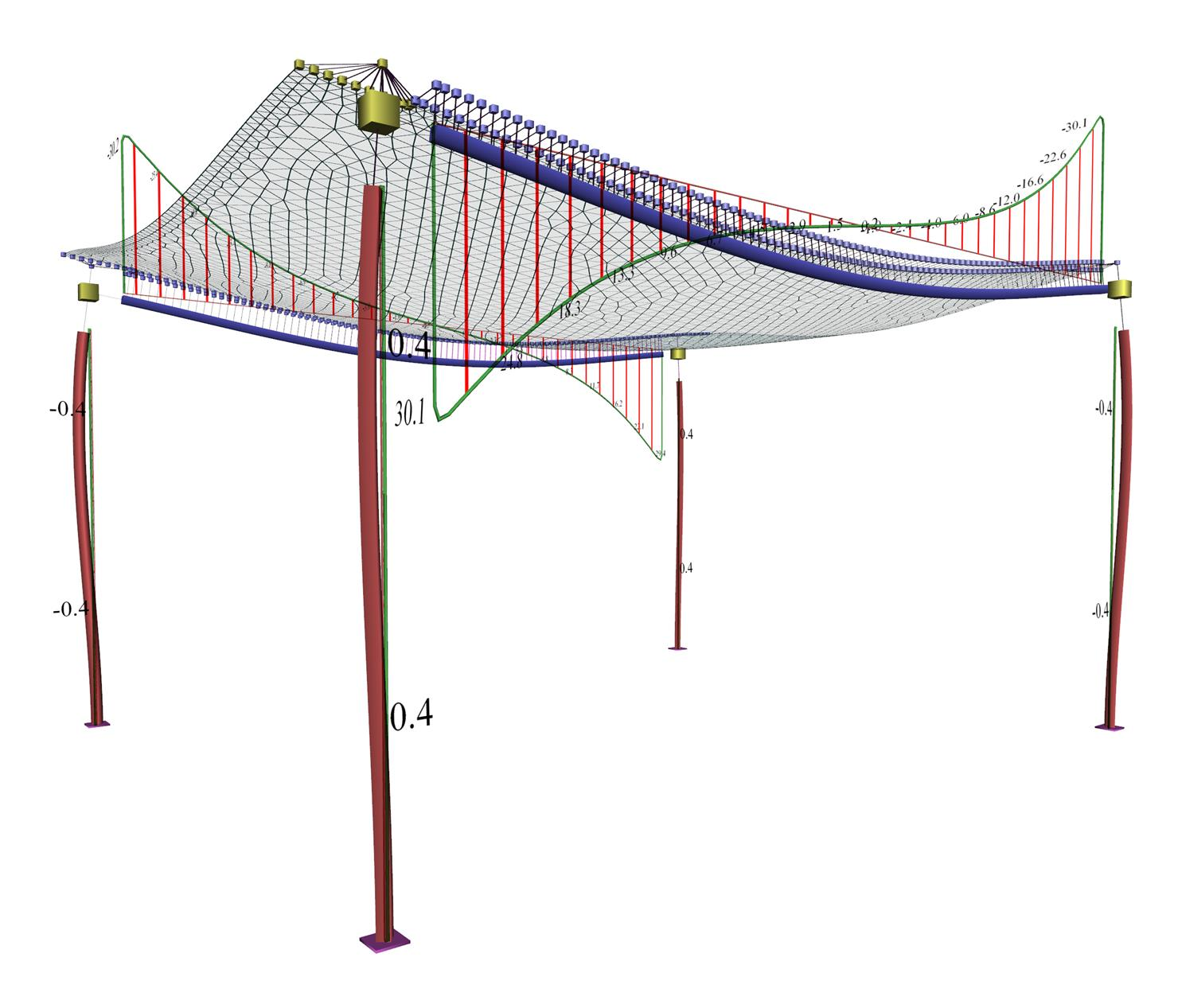Figure 3.3.6.3-4: Torsional moments of beams of torsional stiffness 100%. Triangular finite element model Figure 3.3.6.3-4: Torsional moments of beams of torsional stiffness 100%. Triangular finite element model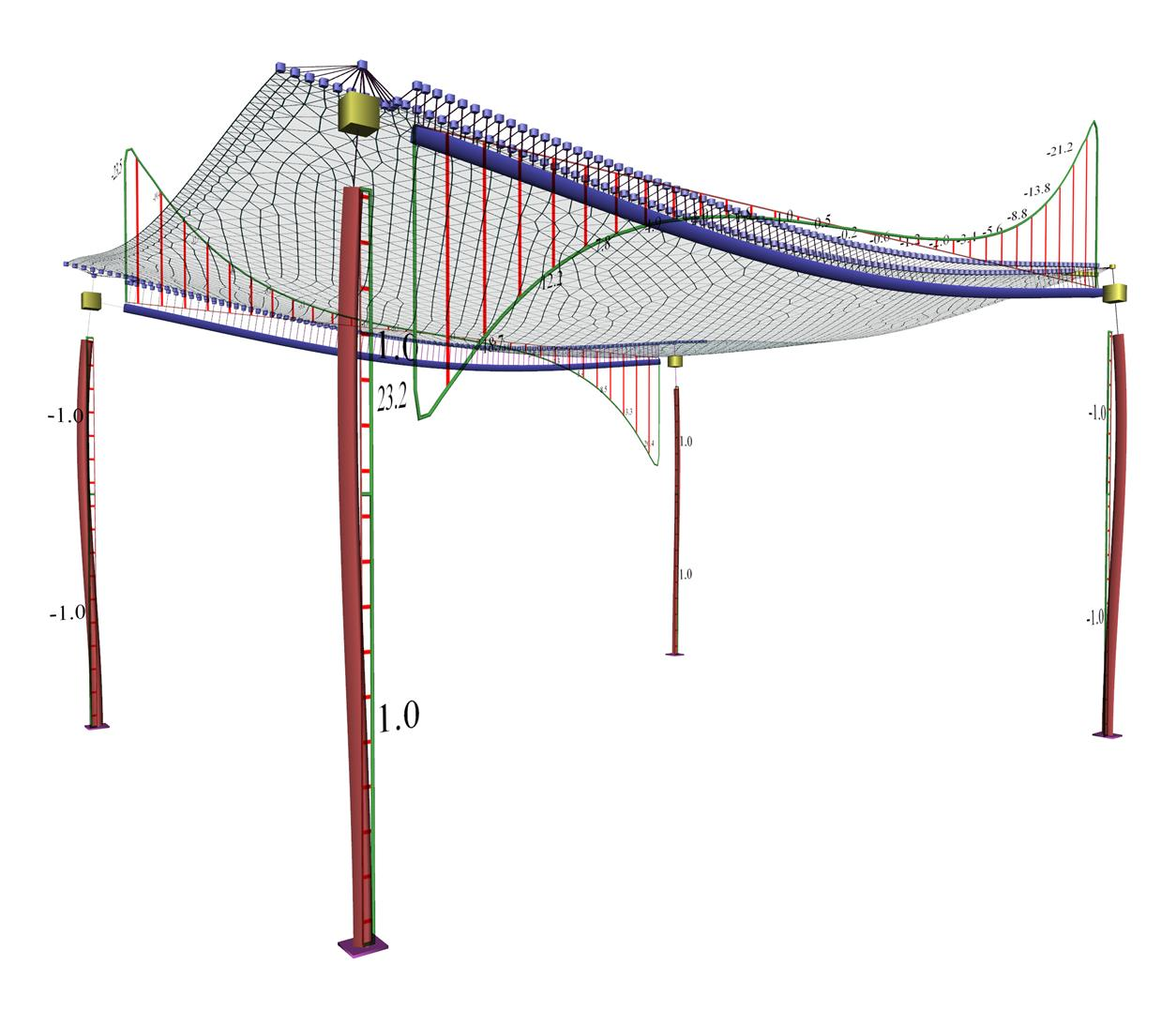Figure 3.3.6.3-5 Torsional moments of beams of torsional stiffness 10%. Triangular finite element model Figure 3.3.6.3-5 Torsional moments of beams of torsional stiffness 10%. Triangular finite element model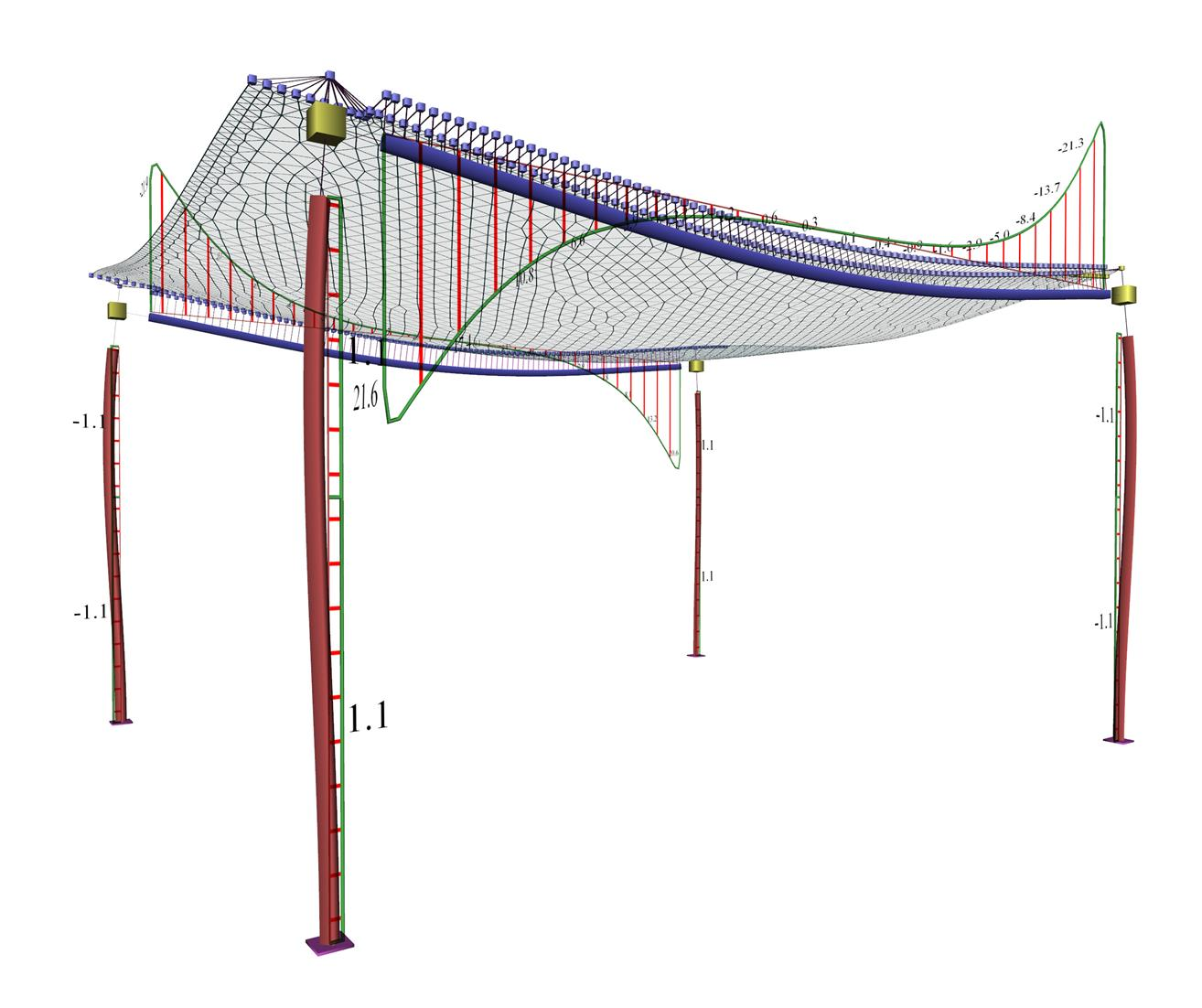Figure 3.3.6.3-6: Torsional moments of beams of torsional stiffness 1%. Triangular finite element model Figure 3.3.6.3-6: Torsional moments of beams of torsional stiffness 1%. Triangular finite element model

« Vertical deformation of beams Final conclusion »